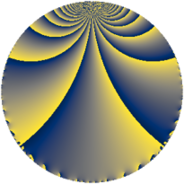# Properties

 Label 4900.2.e.hLevel $4900$ Weight $2$ Character orbit 4900.e Analytic conductor $39.127$ Analytic rank $0$ Dimension $2$ CM no Inner twists $2$

# Related objects

## Newspace parameters

 Level: $$N$$ $$=$$ $$4900 = 2^{2} \cdot 5^{2} \cdot 7^{2}$$ Weight: $$k$$ $$=$$ $$2$$ Character orbit: $$[\chi]$$ $$=$$ 4900.e (of order $$2$$, degree $$1$$, not minimal)

## Newform invariants

 Self dual: no Analytic conductor: $$39.1266969904$$ Analytic rank: $$0$$ Dimension: $$2$$ Coefficient field: $$\Q(\sqrt{-1})$$ Defining polynomial: $$x^{2} + 1$$ Coefficient ring: $$\Z[a_1, a_2, a_3]$$ Coefficient ring index: $$1$$ Twist minimal: no (minimal twist has level 28) Sato-Tate group: $\mathrm{SU}(2)[C_{2}]$

## $q$-expansion

Coefficients of the $$q$$-expansion are expressed in terms of $$i = \sqrt{-1}$$. We also show the integral $$q$$-expansion of the trace form.

 $$f(q)$$ $$=$$ $$q + i q^{3} + 2 q^{9} +O(q^{10})$$ $$q + i q^{3} + 2 q^{9} -3 q^{11} + 2 i q^{13} -3 i q^{17} - q^{19} -3 i q^{23} + 5 i q^{27} + 6 q^{29} + 7 q^{31} -3 i q^{33} -i q^{37} -2 q^{39} -6 q^{41} + 4 i q^{43} + 9 i q^{47} + 3 q^{51} -3 i q^{53} -i q^{57} + 9 q^{59} + q^{61} -7 i q^{67} + 3 q^{69} -i q^{73} + 13 q^{79} + q^{81} + 12 i q^{83} + 6 i q^{87} + 15 q^{89} + 7 i q^{93} + 10 i q^{97} -6 q^{99} +O(q^{100})$$ $$\operatorname{Tr}(f)(q)$$ $$=$$ $$2q + 4q^{9} + O(q^{10})$$ $$2q + 4q^{9} - 6q^{11} - 2q^{19} + 12q^{29} + 14q^{31} - 4q^{39} - 12q^{41} + 6q^{51} + 18q^{59} + 2q^{61} + 6q^{69} + 26q^{79} + 2q^{81} + 30q^{89} - 12q^{99} + O(q^{100})$$

## Character values

We give the values of $$\chi$$ on generators for $$\left(\mathbb{Z}/4900\mathbb{Z}\right)^\times$$.

 $$n$$ $$101$$ $$1177$$ $$2451$$ $$\chi(n)$$ $$1$$ $$-1$$ $$1$$

## Embeddings

For each embedding $$\iota_m$$ of the coefficient field, the values $$\iota_m(a_n)$$ are shown below.

For more information on an embedded modular form you can click on its label.

Label $$\iota_m(\nu)$$ $$a_{2}$$ $$a_{3}$$ $$a_{4}$$ $$a_{5}$$ $$a_{6}$$ $$a_{7}$$ $$a_{8}$$ $$a_{9}$$ $$a_{10}$$
2549.1
 − 1.00000i 1.00000i
0 1.00000i 0 0 0 0 0 2.00000 0
2549.2 0 1.00000i 0 0 0 0 0 2.00000 0
 $$n$$: e.g. 2-40 or 990-1000 Significant digits: Format: Complex embeddings Normalized embeddings Satake parameters Satake angles

## Inner twists

Char Parity Ord Mult Type
1.a even 1 1 trivial
5.b even 2 1 inner

## Twists

By twisting character orbit
Char Parity Ord Mult Type Twist Min Dim
1.a even 1 1 trivial 4900.2.e.h 2
5.b even 2 1 inner 4900.2.e.h 2
5.c odd 4 1 196.2.a.a 1
5.c odd 4 1 4900.2.a.n 1
7.b odd 2 1 4900.2.e.i 2
7.d odd 6 2 700.2.r.b 4
15.e even 4 1 1764.2.a.j 1
20.e even 4 1 784.2.a.g 1
35.c odd 2 1 4900.2.e.i 2
35.f even 4 1 196.2.a.b 1
35.f even 4 1 4900.2.a.g 1
35.i odd 6 2 700.2.r.b 4
35.k even 12 2 28.2.e.a 2
35.k even 12 2 700.2.i.c 2
35.l odd 12 2 196.2.e.a 2
40.i odd 4 1 3136.2.a.v 1
40.k even 4 1 3136.2.a.k 1
60.l odd 4 1 7056.2.a.bw 1
105.k odd 4 1 1764.2.a.a 1
105.w odd 12 2 252.2.k.c 2
105.x even 12 2 1764.2.k.b 2
140.j odd 4 1 784.2.a.d 1
140.w even 12 2 784.2.i.d 2
140.x odd 12 2 112.2.i.b 2
280.s even 4 1 3136.2.a.h 1
280.y odd 4 1 3136.2.a.s 1
280.bp odd 12 2 448.2.i.c 2
280.bv even 12 2 448.2.i.e 2
315.bs even 12 2 2268.2.i.a 2
315.bu odd 12 2 2268.2.i.h 2
315.bw odd 12 2 2268.2.l.a 2
315.cg even 12 2 2268.2.l.h 2
420.w even 4 1 7056.2.a.f 1
420.br even 12 2 1008.2.s.p 2

By twisted newform orbit
Twist Min Dim Char Parity Ord Mult Type
28.2.e.a 2 35.k even 12 2
112.2.i.b 2 140.x odd 12 2
196.2.a.a 1 5.c odd 4 1
196.2.a.b 1 35.f even 4 1
196.2.e.a 2 35.l odd 12 2
252.2.k.c 2 105.w odd 12 2
448.2.i.c 2 280.bp odd 12 2
448.2.i.e 2 280.bv even 12 2
700.2.i.c 2 35.k even 12 2
700.2.r.b 4 7.d odd 6 2
700.2.r.b 4 35.i odd 6 2
784.2.a.d 1 140.j odd 4 1
784.2.a.g 1 20.e even 4 1
784.2.i.d 2 140.w even 12 2
1008.2.s.p 2 420.br even 12 2
1764.2.a.a 1 105.k odd 4 1
1764.2.a.j 1 15.e even 4 1
1764.2.k.b 2 105.x even 12 2
2268.2.i.a 2 315.bs even 12 2
2268.2.i.h 2 315.bu odd 12 2
2268.2.l.a 2 315.bw odd 12 2
2268.2.l.h 2 315.cg even 12 2
3136.2.a.h 1 280.s even 4 1
3136.2.a.k 1 40.k even 4 1
3136.2.a.s 1 280.y odd 4 1
3136.2.a.v 1 40.i odd 4 1
4900.2.a.g 1 35.f even 4 1
4900.2.a.n 1 5.c odd 4 1
4900.2.e.h 2 1.a even 1 1 trivial
4900.2.e.h 2 5.b even 2 1 inner
4900.2.e.i 2 7.b odd 2 1
4900.2.e.i 2 35.c odd 2 1
7056.2.a.f 1 420.w even 4 1
7056.2.a.bw 1 60.l odd 4 1

## Hecke kernels

This newform subspace can be constructed as the intersection of the kernels of the following linear operators acting on $$S_{2}^{\mathrm{new}}(4900, [\chi])$$:

 $$T_{3}^{2} + 1$$ $$T_{11} + 3$$ $$T_{19} + 1$$ $$T_{31} - 7$$

## Hecke characteristic polynomials

$p$ $F_p(T)$
$2$ $$T^{2}$$
$3$ $$1 + T^{2}$$
$5$ $$T^{2}$$
$7$ $$T^{2}$$
$11$ $$( 3 + T )^{2}$$
$13$ $$4 + T^{2}$$
$17$ $$9 + T^{2}$$
$19$ $$( 1 + T )^{2}$$
$23$ $$9 + T^{2}$$
$29$ $$( -6 + T )^{2}$$
$31$ $$( -7 + T )^{2}$$
$37$ $$1 + T^{2}$$
$41$ $$( 6 + T )^{2}$$
$43$ $$16 + T^{2}$$
$47$ $$81 + T^{2}$$
$53$ $$9 + T^{2}$$
$59$ $$( -9 + T )^{2}$$
$61$ $$( -1 + T )^{2}$$
$67$ $$49 + T^{2}$$
$71$ $$T^{2}$$
$73$ $$1 + T^{2}$$
$79$ $$( -13 + T )^{2}$$
$83$ $$144 + T^{2}$$
$89$ $$( -15 + T )^{2}$$
$97$ $$100 + T^{2}$$